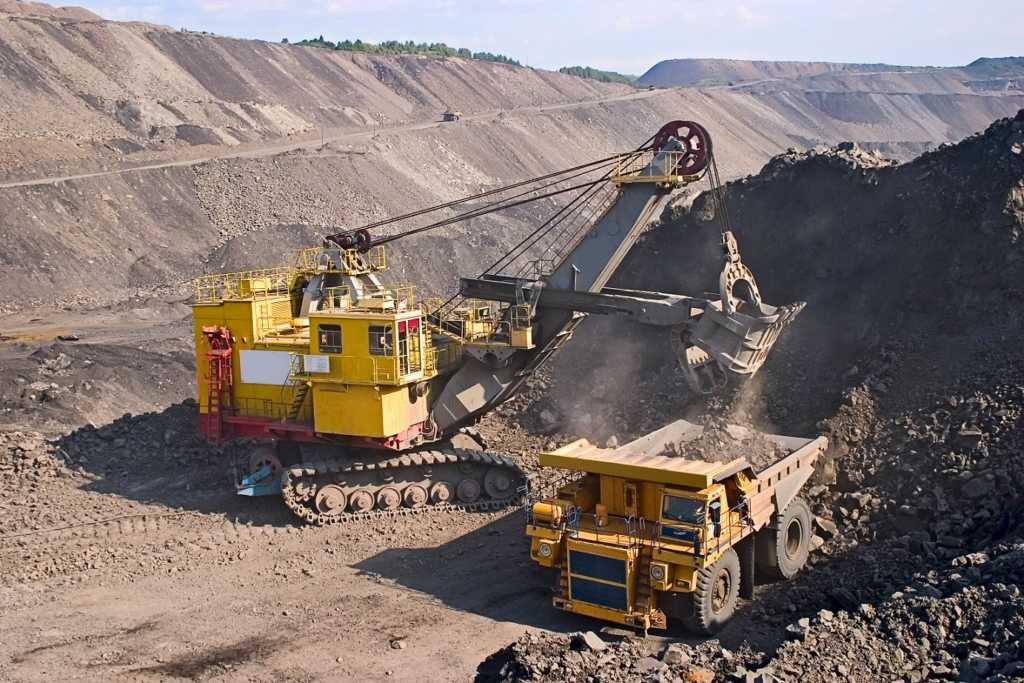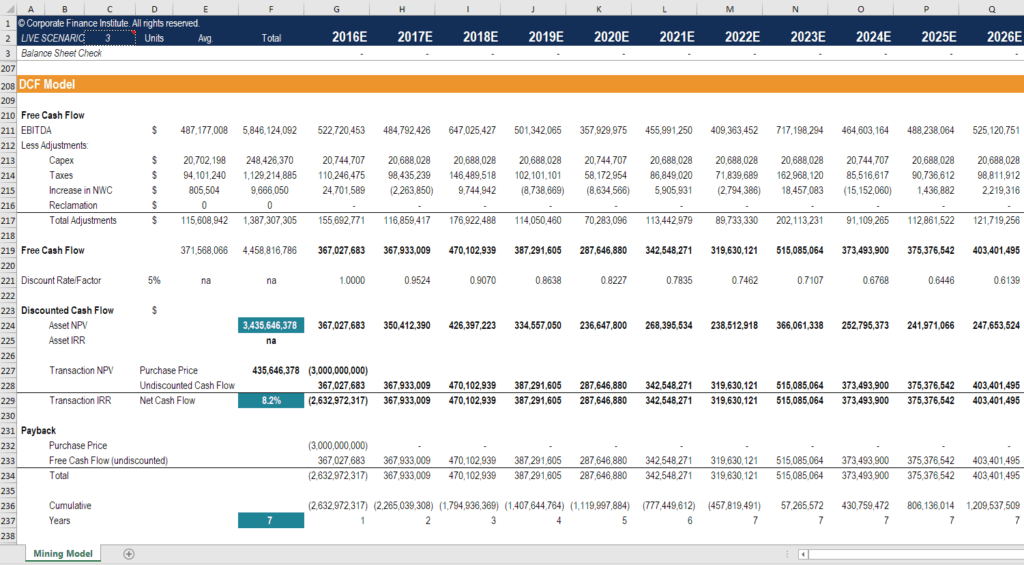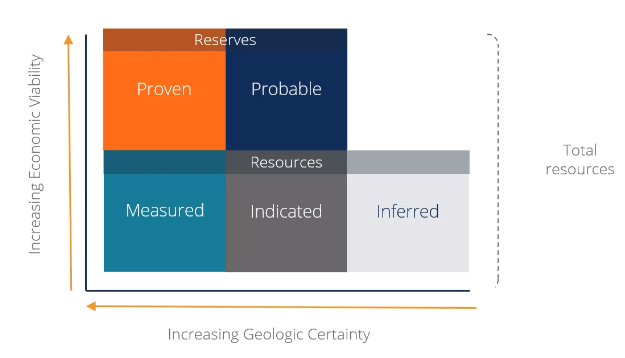# Mining Asset Valuation Techniques

Key valuation metrics used to value mining assets

## How do you value a mining asset?

Mining assets are very challenging to value. Given the degree of geologic uncertainty around reserves and resources, it’s hard to know how much metal is actually in the ground. This guide to mining valuation will teach you all you need to know to value an asset!

The best way to value a mining asset or company is to build a discounted cash flow (DCF) model that takes into account a mining plan produced in a technical report (like a Feasibility Study). Without such a study available, one has to resort to more crude metrics.

Here is an overview of the main valuation methods used in the industry:

• Price to Net Asset Value (P/NAV)
• Price to Cash Flow (P/CF)
• Total Acquisition Cost (TAC)
• EV/Resource (\$/ounce)### P/NAV

Price to net asset value (P/NAV) is the most important mining valuation metric, period.

“Net asset value” is the net present value (NPV) or discounted cash flow (DCF) value of all the future cash flow of the mining asset less any debt plus any cash. The model can be forecast to the end of the mine life and discounted back today because the technical reports have a very detailed Life of Mine plan (LOM).

The formula is as follows:

P/NAV = Market Capitalization / [NPV of all mining assets – net debt]

For a more detailed overview of P/NAV see our course on mining financial modeling.

NAV is a sum-of-the-parts approach to valuation, in that each individual mining asset is independently valued and then added together. Corporate adjustments are made at the end, such as head office overhead or debt.

Below is a screenshot of a NAV model from CFI’s Mining Financial Modeling Course.### P/CF

The price to cash flow ratio, or “P-cash flow” is also common but only used for producing mines, as it takes the current cash flow in that year, relative to the price in the security.

The ratio takes the adjusted cash flow of the business in a given year (i.e. 2018E), and compares that to the share price.

Operating cash flow is after interest (and thus an equity metric) it’s also after taxes, but it does not include capital expenditures.

The formula is as follows:

P/CF = Price per Share / Cash From Operations per Share

We discuss P/CF in more detail in our mining valuation course.

### EV/Resource

This ratio takes the total resources contained in the ground and divides it by the enterprise value of the business.

This metric is typically used for early-stage development projects, where there is not a lot of detailed information (not enough to do a DCF analysis).

The ratio is very basic and doesn’t take into account the capital cost to build the mine, nor the operating cost to extract the metal.

The formula is as follows:

EV/Resource = Enterprise Value / Total Ounces or Pounds of Metal Resource

### TAC (Total Acquisition Cost)

Another commonly used metric in the mining industry for early-stage projects is Total Acquisition Cost or TAC.

This represents the cost to acquire the asset, build the mine and operate the mine, all on a per ounce basis.

An example of calculating TAC:

Suppose that a publicly traded stock, had a market capitalization of \$100 million dollars, and it had \$1 million ounces.

I could, therefore, acquire the asset for \$100 dollars per ounce.

I know that the cost of building the mine divided by the number of ounces would be \$200 dollars per ounce.

I also know that the average all-and-sustaining cost to operate this mine is about \$900 dollars per ounce. Based on some studies.

All this combines for a \$1,200 per ounce TAC.

The formula is as follows:

TAC = [Cost to Acquire + Cost to Build + Cost to Operate] / Total Ounces

For detail on all of the above metrics, take a free trial of our mining financial modeling and valuation course.### More mining valuation resources

This has been a free guide to mining asset valuation techniques.  To keep learning and expanding your knowledge, check out these additional resources:

• Financial modeling guide
• DCF modeling
• Free excel crash course
• Excel shortcuts

### Valuation Techniques

Learn the most important valuation techniques in CFI’s Business Valuation course!

Step by step instruction on how the professionals on Wall Street value a company.

Learn valuation the easy way with templates and step by step instruction!